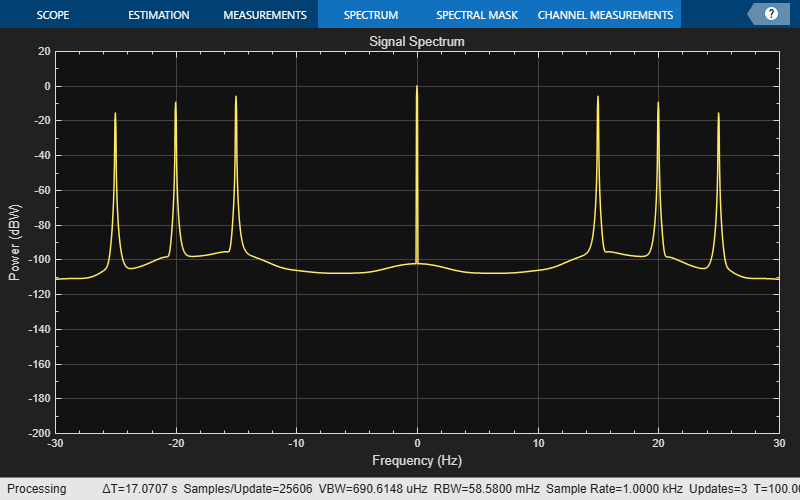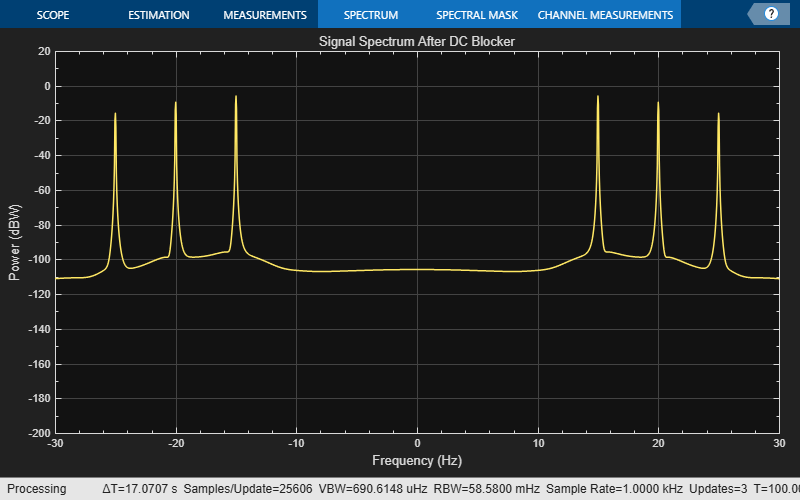Main Content

# dsp.DCBlocker

Block DC component (offset) from input signal

## Description

The `dsp.DCBlocker` System object™ removes the DC offset from each channel (column) of the input signal. The operation runs over time to continually estimate and remove the DC offset.

To block the DC component of the input signal:

1. Create the `dsp.DCBlocker` object and set its properties.

2. Call the object with arguments, as if it were a function.

To learn more about how System objects work, see What Are System Objects?.

## Creation

### Syntax

``dcblker = dsp.DCBlocker``
``dcblker = dsp.DCBlocker(Name,Value)``

### Description

example

````dcblker = dsp.DCBlocker` creates a DC blocker System object, `dcblker`, to block the DC component from each channel (column) of the input signal.```

example

````dcblker = dsp.DCBlocker(Name,Value)` creates a DC blocker System object, `dcblker`, with each specified property set to the specified value. Enclose each property name in single quotes.Example: dcblker = dsp.DCBlocker('Algorithm','FIR')```

## Properties

expand all

Unless otherwise indicated, properties are nontunable, which means you cannot change their values after calling the object. Objects lock when you call them, and the `release` function unlocks them.

If a property is tunable, you can change its value at any time.

For more information on changing property values, see System Design in MATLAB Using System Objects.

Algorithm for estimating DC offset, specified as one of the following:

• `'IIR'` –– The object uses a recursive estimate based on a narrow, lowpass elliptic filter. The Order property sets the order of the filter, and the NormalizedBandwidth property sets its bandwidth. This algorithm typically uses less memory than the FIR algorithm and is more efficient.

• `'FIR'` –– The object uses a nonrecursive, moving average estimate based on a finite number of past input samples. The Length property sets the number of samples. The FIR filter has a linear phase response and does not cause any phase distortion to the signal. The IIR filter requires less memory and is more efficient to implement.

• `'CIC'` –– The object uses a CIC decimator, with a decimation factor of 1, whose differential delay is calculated using the NormalizedBandwidth property. It uses two sections to ensure that the first sidelobe attenuation is at least 25 dB below the main lobe of the filter. This algorithm requires fixed-point inputs and can be used for HDL code generation.

• `'Subtract mean'` –– The object computes the means of the columns of the input matrix, and subtracts the means from the input. This method does not retain state between inputs.

You can visualize the IIR, FIR, and CIC responses by using the `fvtool` function.

Normalized bandwidth of the IIR or CIC filter, specified as a real scalar greater than 0 or less than 1. The normalized bandwidth is used to estimate the DC component of the input signal.

#### Dependencies

This property applies only when you set the Algorithm property to `'IIR'` or `'CIC'`.

Order of the lowpass IIR elliptic filter that is used to estimate the DC level, specified as an integer greater than 3.

#### Dependencies

This property applies only when you set the Algorithm property to `'IIR'`.

Number of past input samples used in the FIR algorithm to estimate the running mean, specified as a positive integer.

#### Dependencies

This property applies only when you set the Algorithm property to `'FIR'`.

## Usage

### Syntax

``dcblkerOut = dcblker(input)``

### Description

example

````dcblkerOut = dcblker(input)` removes the DC component from each channel (column) of the input and returns the output.```

### Input Arguments

expand all

Data input to the DC blocker object, specified as a vector, matrix, or N-D array.

Example: t = (0:0.001:100)'; x = sin(30*pi*t) + 1;

Data Types: `single` | `double` | `int8` | `int16` | `int32` | `fi`
Complex Number Support: Yes

### Output Arguments

expand all

Signal with DC component removed, returned as a vector, matrix, or N-D array.The output dimensions match the input dimensions.

Data Types: `single` | `double` | `int8` | `int16` | `int32` | `fi`
Complex Number Support: Yes

## Object Functions

To use an object function, specify the System object as the first input argument. For example, to release system resources of a System object named `obj`, use this syntax:

`release(obj)`

expand all

 `fvtool` Visualize frequency response of DSP filters
 `step` Run System object algorithm `release` Release resources and allow changes to System object property values and input characteristics `reset` Reset internal states of System object

## Examples

collapse all

Note: If you are using R2016a or earlier, replace each call to the object with the equivalent step syntax. For example, obj(x) becomes step(obj,x).

Remove the DC component of an input signal using the IIR, FIR, and subtract mean estimation algorithms.

Create a signal composed of a 15 Hz tone, a 25 Hz tone, and a DC offset.

```t = (0:0.001:100)'; x = sin(30*pi*t) + 0.33*cos(50*pi*t) + 1;```

Create three DC blocker objects for the three estimation algorithms.

```dc1 = dsp.DCBlocker('Algorithm','IIR','Order', 6); dc2 = dsp.DCBlocker('Algorithm','FIR','Length', 100); dc3 = dsp.DCBlocker('Algorithm','Subtract mean');```

For each second of time, pass the input signal through the DC blockers. By implementing the DC blockers in 1-second increments, you can observe differences in the convergence times.

```for idx = 1 : 100 range = (1:1000) + 1000*(idx-1); y1 = dc1(x(range)); % IIR estimate y2 = dc2(x(range)); % FIR estimate y3 = dc3(x(range)); % Subtract mean end```

Plot the input and output data for the three DC blockers for the first second of time, and show the mean value for each signal. The mean values for the three algorithm types show that the `FIR` and `Subtract mean` algorithms converge more quickly.

```plot(t(1:1000),x(1:1000), ... t(1:1000),y1, ... t(1:1000),y2, ... t(1:1000),y3); xlabel('Time (sec)') ylabel('Amplitude') legend(sprintf('Input DC:%.3f',mean(x)), ... sprintf('IIR DC:%.3f',mean(y1)), ... sprintf('FIR DC:%.3f',mean(y2)), ... sprintf('Subtract mean DC:%.3f',mean(y3)));```Note: If you are using R2016a or earlier, replace each call to the object with the equivalent step syntax. For example, obj(x) becomes step(obj,x).

Compare the spectrum of an input signal with a DC offset to the spectrum of the same signal after applying a DC blocker. Enable the DC blocker to use the `FIR` estimation algorithm.

Create an input signal composed of three tones and that has a DC offset of 1. Set the sampling frequency to 1 kHz and set the signal duration to 100 seconds.

```fs = 1000; t = (0:1/fs:100)'; x = sin(30*pi*t) + 0.67*sin(40*pi*t) + 0.33*sin(50*pi*t) + 1; ```

Create a DC blocker object that uses the FIR algorithm to estimate the DC offset.

```dcblker = dsp.DCBlocker('Algorithm','FIR','Length',100); ```

Create a spectrum analyzer with power units set to `dBW` and a frequency range of `[-30 30]` to display the frequency response of the input signal. Using the `clone` function, create a second spectrum analyzer to display the response of the output. Then, use the `Title` property of the spectrum analyzers to label them.

```hsa = dsp.SpectrumAnalyzer('SampleRate',fs, ... 'PowerUnits','dBW','FrequencySpan','Start and stop frequencies',... 'StartFrequency',-30,'StopFrequency',30,'YLimits',[-200 20],... 'Title','Signal Spectrum'); hsb = clone(hsa); hsb.Title = 'Signal Spectrum After DC Blocker'; ```

Pass the input signal, `x`, through the DC blocker to generate the output signal, `y`.

```y = dcblker(x); ```

Use the first spectrum analyzer to display the frequency characteristics of the input signal. The tones at 15, 20, and 25 Hz, and the DC component, are clearly visible.

```hsa(x) ```Use the second spectrum analyzer to display the frequency characteristics of the output signal. The DC component has been removed.

```hsb(y) ```## Algorithms

expand all

The DC blocker subtracts the DC component from the input signal. You can estimate the DC component by using the IIR, FIR, CIC, or subtract mean algorithm.

 Nezami, M. “Performance Assessment of Baseband Algorithms for Direct Conversion Tactical Software Defined Receivers: I/Q Imbalance Correction, Image Rejection, DC Removal, and Channelization.” MILCOM, 2002.

Watch now